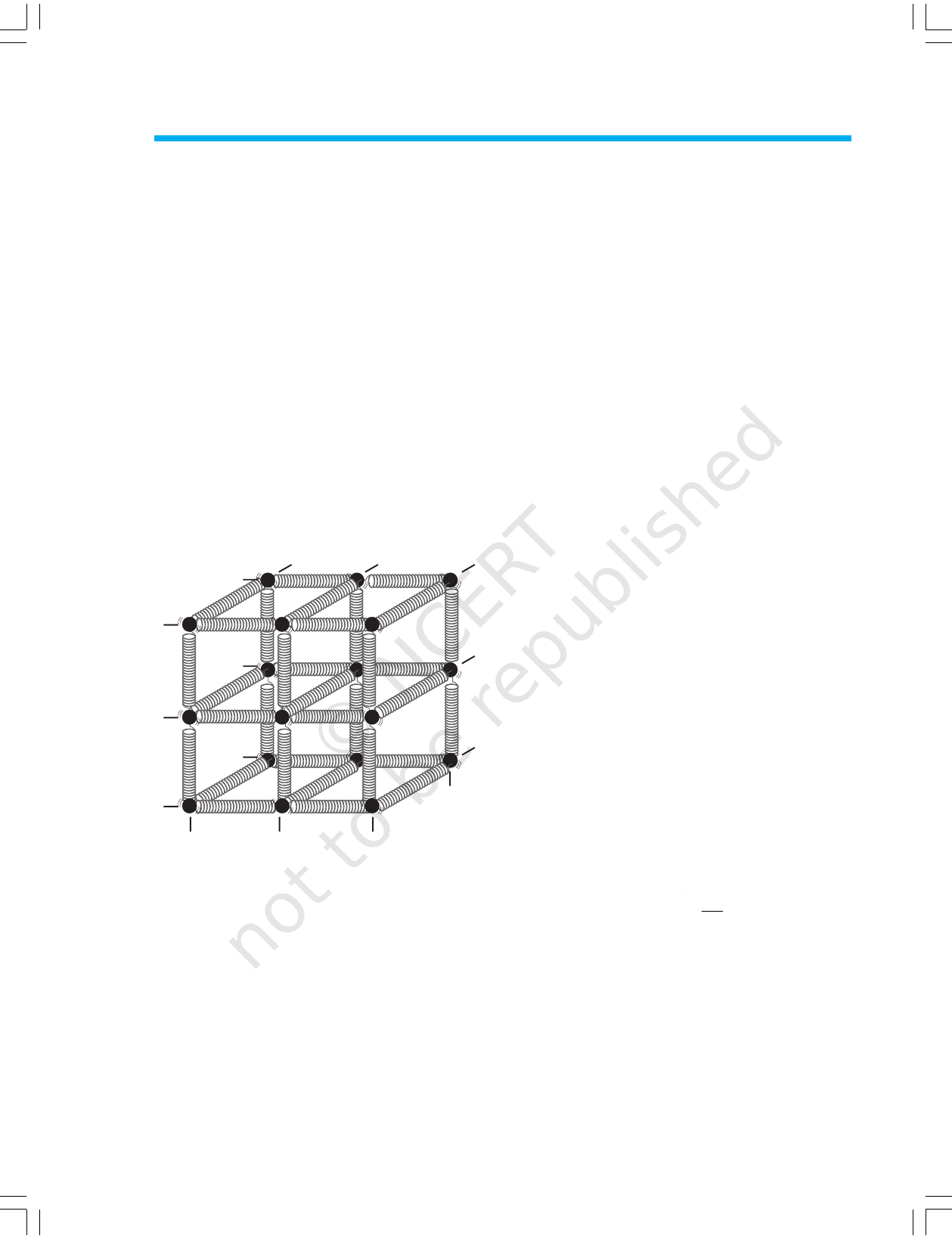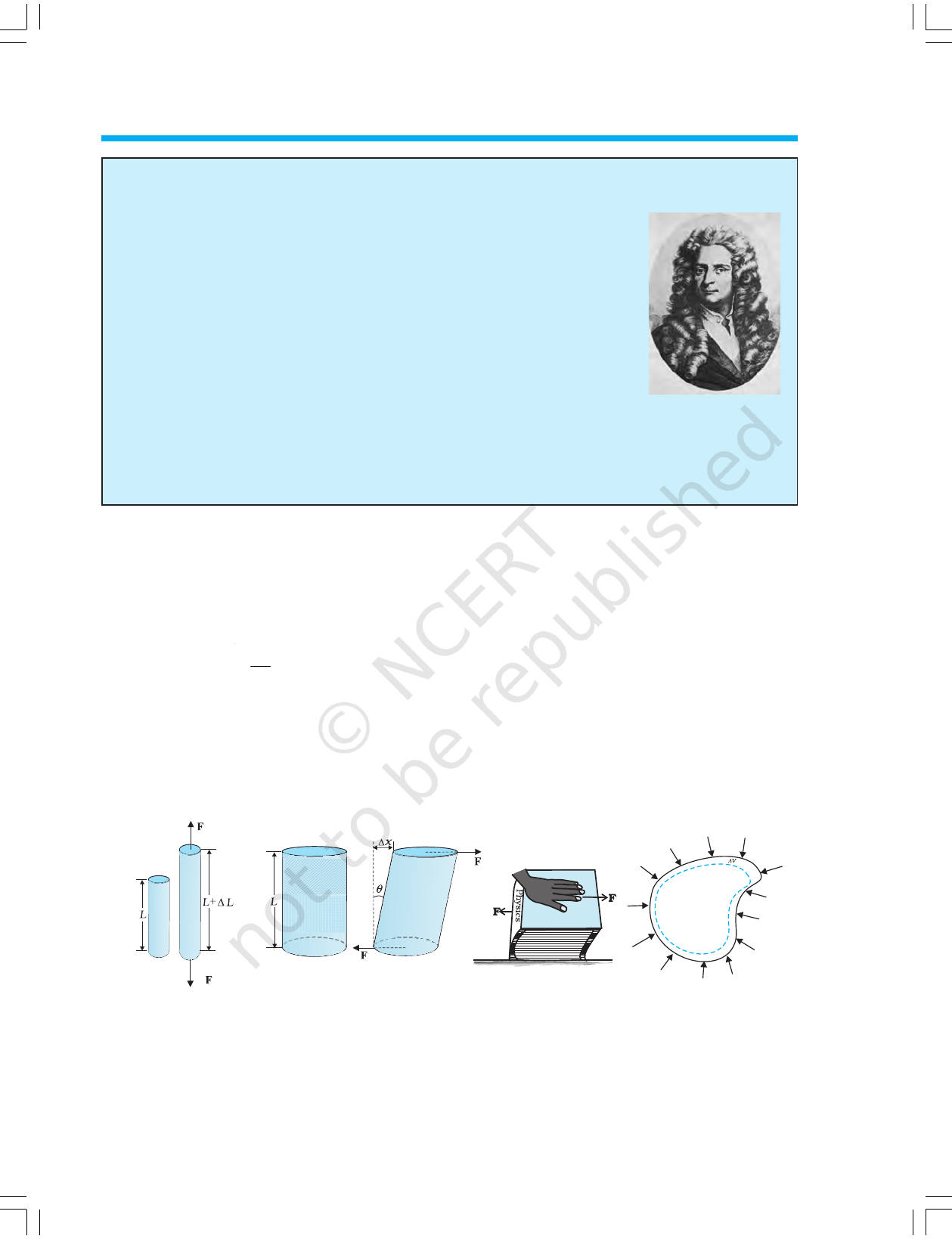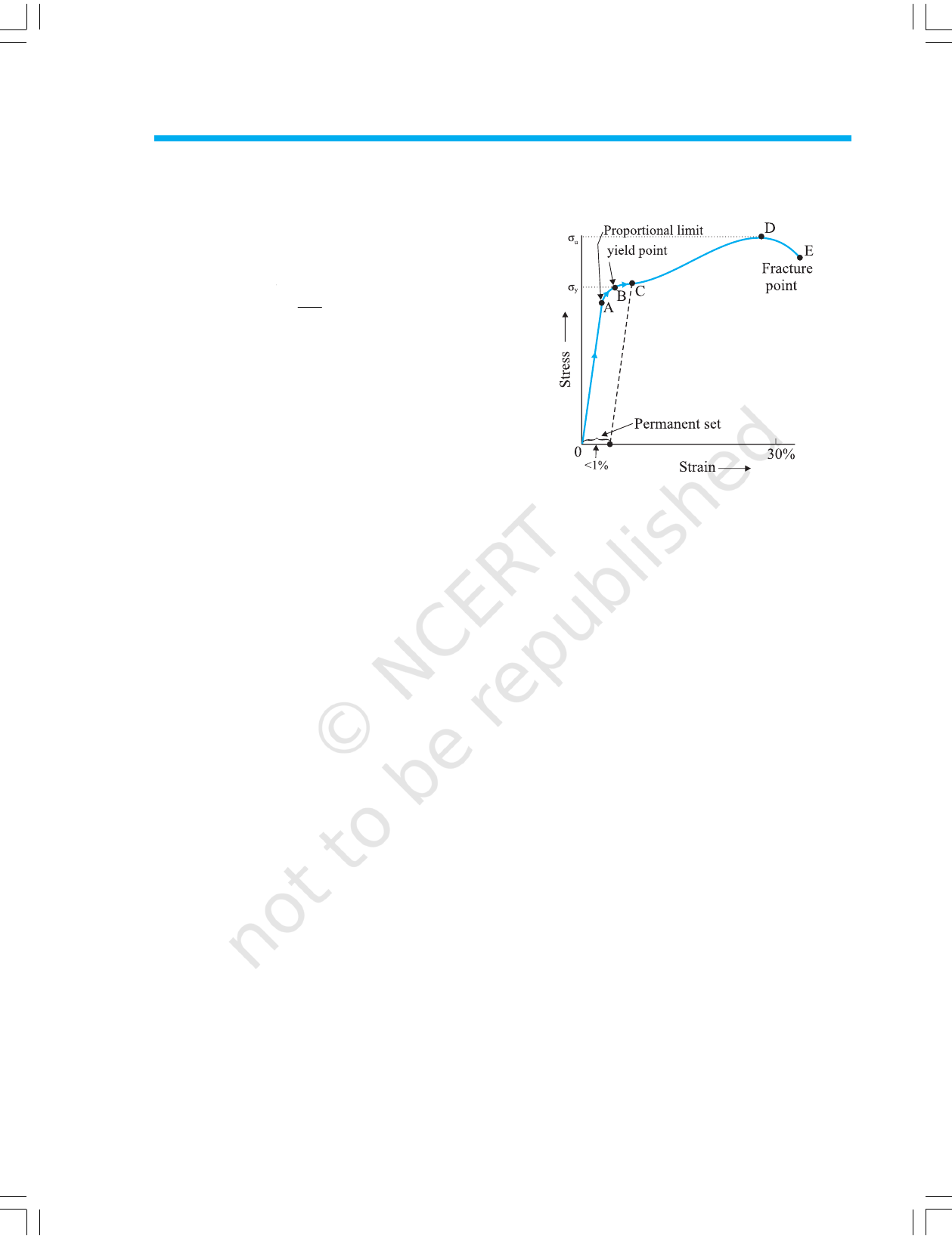CHAPTER NINE
MECHANICAL PROPERTIES OF SOLIDS
9.1 INTRODUCTION
In Chapter 7, we studied the rotation of the bodies and then
realised that the motion of a body depends on how mass is
distributed within the body. We restricted ourselves to simpler
situations of rigid bodies. A rigid body generally means a
hard solid object having a definite shape and size. But in
reality, bodies can be stretched, compressed and bent. Even
the appreciably rigid steel bar can be deformed when a
sufficiently large external force is applied on it. This means
that solid bodies are not perfectly rigid.
A solid has definite shape and size. In order to change (or
deform) the shape or size of a body, a force is required. If
you stretch a helical spring by gently pulling its ends, the
length of the spring increases slightly. When you leave the
ends of the spring, it regains its original size and shape. The
property of a body, by virtue of which it tends to regain its
original size and shape when the applied force is removed, is
known as elasticity and the deformation caused is known
as elastic deformation. However, if you apply force to a lump
of putty or mud, they have no gross tendency to regain their
previous shape, and they get permanently deformed. Such
substances are called plastic and this property is called
plasticity. Putty and mud are close to ideal plastics.
The elastic behaviour of materials plays an important role
in engineering design. For example, while designing a
building, knowledge of elastic properties of materials like steel,
concrete etc. is essential. The same is true in the design of
bridges, automobiles, ropeways etc. One could also ask —
Can we design an aeroplane which is very light but
sufficiently strong? Can we design an artificial limb which
is lighter but stronger? Why does a railway track have a
particular shape like I? Why is glass brittle while brass is
not? Answers to such questions begin with the study of how
relatively simple kinds of loads or forces act to deform
different solids bodies. In this chapter, we shall study the
9.1 Introduction
9.2 Elastic behaviour of solids
9.3 Stress and strain
9.4 Hooke’s law
9.5 Stress-strain curve
9.6 Elastic moduli
9.7 Applications of elastic
behaviour of materials
Summary
Points to ponder
Exercises
2020-21236 PHYSICS
elastic behaviour and mechanical properties of
solids which would answer many such
questions.
9.2 ELASTIC BEHAVIOUR OF SOLIDS
We know that in a solid, each atom or molecule
is surrounded by neighbouring atoms or
molecules. These are bonded together by
interatomic or intermolecular forces and stay
in a stable equilibrium position. When a solid is
deformed, the atoms or molecules are displaced
from their equilibrium positions causing a
change in the interatomic (or intermolecular)
distances. When the deforming force is removed,
the interatomic forces tend to drive them back
to their original positions. Thus the body regains
its original shape and size. The restoring
mechanism can be visualised by taking a model
of spring-ball system shown in the Fig. 9.1. Here
the balls represent atoms and springs represent
interatomic forces.
Fig. 9.1 Spring-ball model for the illustration of elastic
behaviour of solids.
If you try to displace any ball from its
equilibrium position, the spring system tries to
restore the ball back to its original position. Thus
elastic behaviour of solids can be explained in
terms of microscopic nature of the solid. Robert
Hooke, an English physicist (1635 - 1703 A.D)
performed experiments on springs and found
that the elongation (change in the length)
produced in a body is proportional to the applied
force or load. In 1676, he presented his law of
elasticity, now called Hooke’s law. We shall
study about it in Section 9.4. This law, like
Boyle’s law, is one of the earliest quantitative
relationships in science. It is very important to
know the behaviour of the materials under
om the context of
engineering design.
9.3 STRESS AND STRAIN
When forces are applied on a body in such a
manner that the body is still in static equilibrium,
it is deformed to a small or large extent depending
upon the nature of the material of the body and
the magnitude of the deforming force. The
deformation may not be noticeable visually in
many materials but it is there. When a body is
subjected to a deforming force, a restoring force
is developed in the body. This restoring force is
equal in magnitude but opposite in direction to
the applied force. The restoring force per unit area
is known as stress. If F is the force applied normal
to the cross–section and A is the area of cross
section of the body,
Magnitude of the stress = F/A (9.1)
The SI unit of stress is N m
–2
or pascal (Pa)
and its dimensional formula is [ ML
–1
T
–2
].
There are three ways in which a solid may
change its dimensions when an external force
acts on it. These are shown in Fig. 9.2. In
Fig.9.2(a), a cylinder is stretched by two equal
forces applied normal to its cross-sectional area.
The restoring force per unit area in this case
is called tensile stress. If the cylinder is
compressed under the action of applied forces,
the restoring force per unit area is known as
compressive stress. Tensile or compressive
stress can also be termed as longitudinal stress.
In both the cases, there is a change in the
length of the cylinder. The change in the length
L to the original length L of the body (cylinder
in this case) is known as longitudinal strain.
Longitudinal strain
=
L
L
(9.2)
However, if two equal and opposite deforming
forces are applied parallel to the cross-sectional
area of the cylinder, as shown in Fig. 9.2(b),
there is relative displacement between the
opposite faces of the cylinder. The restoring force
per unit area developed due to the applied
tangential force is known as tangential or
shearing stress.
2020-21MECHANICAL PROPERTIES OF SOLIDS 237
As a result of applied tangential force, there
is a relative displacement x between opposite
faces of the cylinder as shown in the Fig. 9.2(b).
The strain so produced is known as shearing
strain and it is defined as the ratio of relative
displacement of the faces x to the length of
the cylinder L.
Shearing strain
=
L
= tan
θ
(9.3)
where
θ
is the angular displacement of the
cylinder from the vertical (original position of
the cylinder). Usually
θ
is very small, tan
θ
is nearly equal to angle
θ
, (if
θ
= 10°, for
example, there is only 1% difference between
θ
and tan
θ
).
It can also be visualised, when a book is
pressed with the hand and pushed horizontally,
as shown in Fig. 9.2 (c).
Thus, shearing strain = tan
θ
θ
(9.4)
In Fig. 9.2 (d), a solid sphere placed in the
fluid under high pressure is compressed
uniformly on all sides. The force applied by the
fluid acts in perpendicular direction at each
point of the surface and the body is said to be
under hydraulic compression. This leads to
decrease in its volume without any change of
its geometrical shape.
The body develops internal restoring forces
that are equal and opposite to the forces applied
by the fluid (the body restores its original shape
and size when taken out from the fluid). The
internal restoring force per unit area in this case
Robert Hooke
(1635 – 1703 A.D.)
Robert Hooke was born on July 18, 1635 in Freshwater, Isle of Wight. He was
one of the most brilliant and versatile seventeenth century English scientists.
He attended Oxford University but never graduated. Yet he was an extremely
talented inventor, instrument-maker and building designer. He assisted Robert
Boyle in the construction of Boylean air pump. In 1662, he was appointed as
Curator of Experiments to the newly founded Royal Society. In 1665, he became
Professor of Geometry in Gresham College where he carried out his astronomi-
cal observations. He built a Gregorian reflecting telescope; discovered the fifth
star in the trapezium and an asterism in the constellation Orion; suggested that
Jupiter rotates on its axis; plotted detailed sketches of Mars which were later
used in the 19
th
century to determine the planet’s rate of rotation; stated the
inverse square law to describe planetary motion, which Newton modified later
etc. He was elected Fellow of Royal Society and also served as the Society’s
Secretary from 1667 to 1682. In his series of observations presented in Micrographia, he suggested
wave theory of light and first used the word ‘cell’ in a biological context as a result of his studies of cork.
Robert Hooke is best known to physicists for his discovery of law of elasticity: Ut tensio, sic vis (This
is a Latin expression and it means as the distortion, so the force). This law laid the basis for studies of
stress and strain and for understanding the elastic materials.
(a) (b) (c) (d)
Fig. 9.2 (a) A cylindrical body under tensile stress elongates by
L (b) Shearing stress on a cylinder deforming it by
an angle
θ
(c) A body subjected to shearing stress (d) A solid body under a stress normal to the surface at
every point (hydraulic stress). The volumetric strain is
V/V, but there is no change in shape.
2020-21238 PHYSICS
is known as hydraulic stress and in magnitude
is equal to the hydraulic pressure (applied force
per unit area).
The strain produced by a hydraulic pressure
is called volume strain and is defined as the
ratio of change in volume (V) to the original
volume (V
).
Volume strain
=
V
V
(9.5)
Since the strain is a ratio of change in
dimension to the original dimension, it has no
units or dimensional formula.
9.4 HOOKE’S LAW
Stress and strain take different forms in the
situations depicted in the Fig. (9.2). For small
deformations the stress and strain are
proportional to each other. This is known as
Hooke’s law.
Thus,
stress strain
stress = k × strain (9.6)
where k is the proportionality constant and is
known as modulus of elasticity.
Hooke’s law is an empirical law and is found
to be valid for most materials. However, there
are some materials which do not exhibit this
linear relationship.
9.5 STRESS-STRAIN CURVE
The relation between the stress and the strain
for a given material under tensile stress can be
found experimentally. In a standard test of
tensile properties, a test cylinder or a wire is
stretched by an applied force. The fractional
change in length (the strain) and the applied
force needed to cause the strain are recorded.
The applied force is gradually increased in steps
and the change in length is noted. A graph is
plotted between the stress (which is equal in
magnitude to the applied force per unit area)
and the strain produced. A typical graph for a
metal is shown in Fig. 9.3. Analogous graphs
for compression and shear stress may also be
obtained. The stress-strain curves vary from
material to material. These curves help us to
understand how a given material deforms with
increasing loads. From the graph, we can see
that in the region between O to A, the curve is
linear. In this region, Hooke’s law is obeyed.
The body regains its original dimensions when
the applied force is removed. In this region, the
solid behaves as an elastic body.
In the region fr